• 多元多项式回归
2021-01-14 00:33:55

在python中进行多元多项式回归的简单方法是什么？在

比方说，我们有N个样本，每个样本有3个特征，每个样本有40个(当然，也可以是任何数字，但在我的例子中是40个)响应变量。我们要做一个函数，把3个自变量和40个响应变量联系起来。为此，我们在N-1个样本上训练一个多项式模型，并估计剩余一个样本的40个响应变量。自变量(X)和响应变量(y)训练和测试数据的维数：X_train = [(N-1) * 3], y_train = [(N-1) * 40], X_test = [1 * 3], y_test = [1 * 40]

正如我所预期的，这种方法应该产生：

^{pr2}$它是9个系数加上每个样本的一个截距来描述多项式。如果我使用David Maust在2015年提出的方法：import numpy as np import pandas as pd from sklearn.linear_model import LinearRegression from sklearn.pipeline import make_pipeline from sklearn.preprocessing import PolynomialFeatures from sklearn.pipeline import * model = make_pipeline(PolynomialFeatures(degree=2),LinearRegression()) y_poly = model.fit(X_train,y_train) coefficients = model.steps.coef_ intercepts = model.steps.intercept_ coefficients.shape [Output: (40, 10)] 对于每个响应变量，我们最终得到10个系数+一个截距，比我预期的多了一个系数。因此，我不清楚这些系数意味着什么，以及如何组成描述我们的响应变量的多项式。我真的希望StackOverflow能帮到我！希望我能很好地定义我的问题。在 更多相关内容 • 对多维数据执行多元多项式回归。 拟合仅限于具有较小修改选项的标准多项式基。 随意实施术语归约启发式。 该功能在 m.file 中有希望足够详细的解释。 随意发表评论或查询。 不再需要任何额外的工具箱！ 前往...matlab • 多元多项式回归的代码 该代码将多变量多项式方程拟合到多变量输出。 我们首先准备数据如下 wb_train = pd.read_excel（r'C：\ Users \ blah \ training.xlsx'）wb_targets = pd.read_excel（r'C：\ Users \ blahD \...Python • 目前，您有兴趣拟合标准多项式基础，而无需进一步干预这些条款。 内容 如何使用输入 清楚的 load Example.mat reg=MultiPolyRegress(X,Y,2) % Gives you your fit. reg = FitParameters: '-----------------' ... •matlab • 主要介绍了scikit-learn线性回归，多元回归，多项式回归的实现，文中通过示例代码介绍的非常详细，对大家的学习或者工作具有一定的参考学习价值，需要的朋友们下面随着小编来一起学习学习吧 • 多项式回归模型、示例（含数据和代码） 目录 前置知识 Numpy c_函数 问题引入 多项式回归函数 核心思路 示例 数据生成 转化多项式回归训练集 预测和绘图 特征缩放 补充：关于多项式的次数选择 多项式回归虽然不再用直线拟合，但也是线性回归的一种，可以转化为多元线性回归，利用多元线性回归的函数解决。所以请确保熟悉多元线性回归相关知识点：多元线性回归模型_Twilight Sparkle.的博客-CSDN博客 在学习多项式回归之前，你可能需要先了解以下内容： # 前置知识 多项式_百度百科 (baidu.com) 多项式函数_百度百科 (baidu.com) ## Numpy c_函数 该函数可以实现按列拼接矩阵，具体用法： import numpy as np x = np.array([,,,,]) print(x) X = np.c_[x,x**2,x**3] print("np.c_(x,x**2,x**3):") print(X)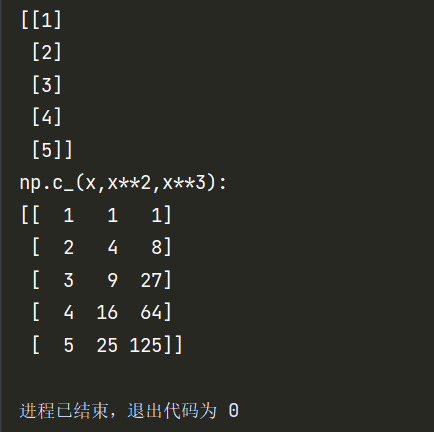# 问题引入 现实生活中呈（狭义）线性关系的事物联系很少，绝大部分都是（狭义）非线性的，即呈曲线形式的关系。所以我们需要引入多项式回归来更好的拟合数据。 # 多项式回归函数 多项式回归又分为多元多项式回归和一元多项式回归： 一元多项式： 多元多项式：这里只举二元二次多项式 不管它是什么多项式，在处理上不同的地方都只是在数据预处理上，核心方法不变。这里为了绘图，以一元多项式进行讲解。 # 核心思路 ,于是多项式回归转化成了多元线性回归。然后套用多元线性回归的函数求解向量和常量即可。 # 示例 注：以下函数均来自上篇文章（多元线性回归） Zscore()：Z-score标准化 gradient_descent()：拟合回归函数,为了更好用，把初始化w和b的函数重新写了一下。 gradient_descent()初始化w，b修改：  m, n = X.shape # initialize parameters init_w = np.zeros(n) init_b = 0 ## 数据生成 以下代码可以生成该示例的训练集数据： def data_generation(): ''' :return: X: 自变量训练集（矩阵） Y: 应变量训练集（一维） ''' x = np.arange(0,40,1) y = 0.25*x**2-0.20*x**3+0.0055*x**4 # 要将X训练集转化为(m,n)的矩阵，即m个例子，n个特征，现在是一元，所以只有一列 x = x.reshape(-1,1) # 生成随机数，稍微打乱y np.random.seed(1) y = y + np.random.random(40)*120 return x,y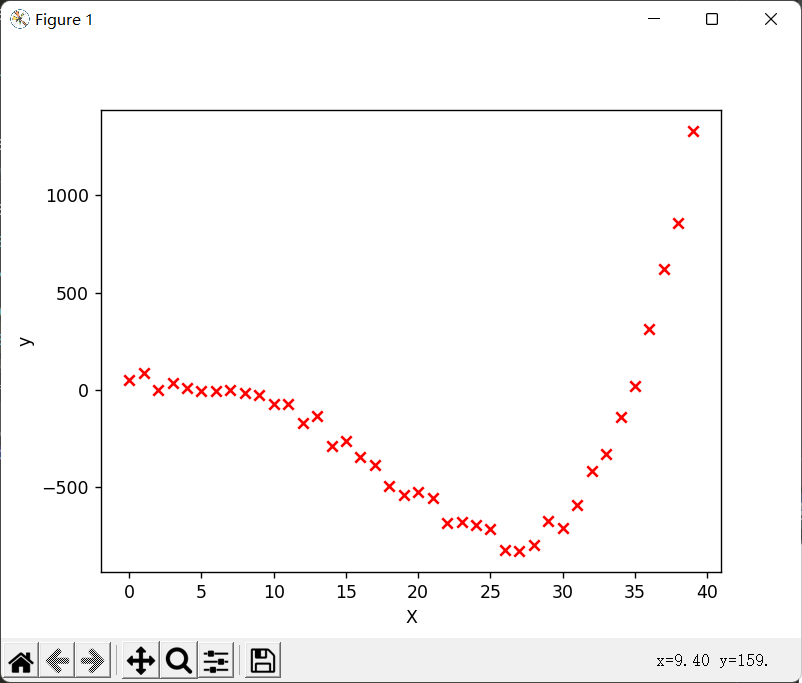## 转化多项式回归训练集 def x_transform(x): ''' :param x:转化前的X训练集 :return: 转化后的X训练集 ''' X_train = np.c_[x,x**2,x**3,x**4] return X_train ## 预测和绘图 if __name__ == '__main__': # 生成数据并绘制训练集图 x_train,y_train = data_generation() plt.scatter(x_train,y_train,marker='x',c='r',label = 'Actual Value') plt.xlabel("X") plt.ylabel("y") # plt.show() # 将x训练集转化为多元线性训练集 x_train_transform = x_transform(x_train) # 预测并绘图 model_w,model_b = gradient_descent(x_train_transform,y_train,alpha=0.000000000003,num_iters=100000) plt.plot(x_train,np.dot(x_train_transform,model_w) + model_b,label="Predicted Value") plt.legend() plt.show() 结果：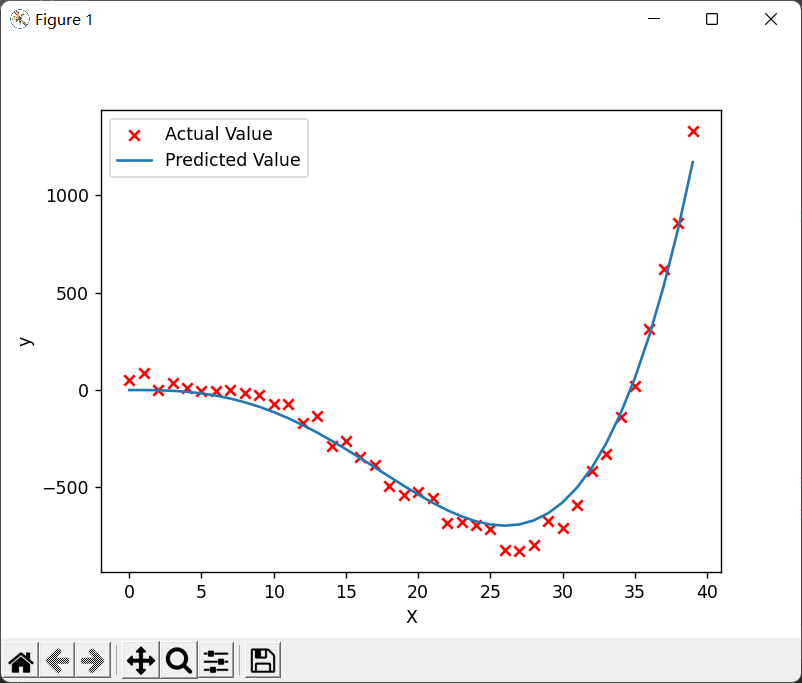## 特征缩放 因为转化为多元一次回归后，会发现每个特征的范围差的特别大（比如），为了照顾取值大的特征，学习率必须设置的非常小，所以梯度下降特别慢。这时，就要用到之前说过的特征缩放了。 特征缩放后的预测和绘图：  # 将x训练集转化为多元线性训练集 x_train_transform = x_transform(x_train) x_train_transform, mu, sigma = Zscore(x_train_transform) # 预测并绘图 model_w,model_b = gradient_descent(x_train_transform,y_train,alpha=0.5,num_iters=10000) plt.plot(x_train,np.dot(x_train_transform,model_w) + model_b,label="Predicted Value") plt.legend() plt.show() 对比学习率和迭代次数，根本不是一个级别的。而且在更短时间内模拟的更好：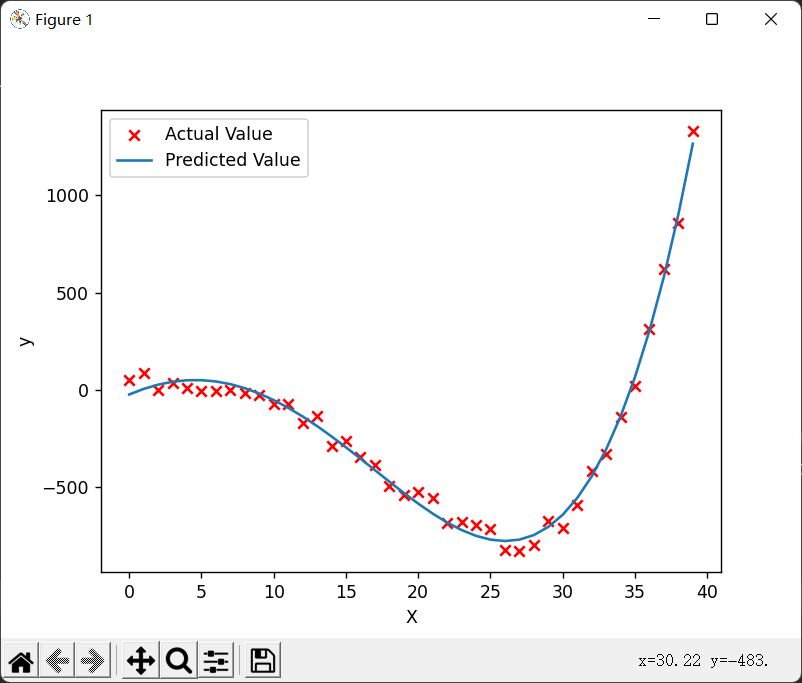所以特征缩放很重要！！ # 补充：关于多项式的次数选择 次数的选择： 多项式函数有多种，一般来说，需要先观察数据的形状，再去决定选用什么形式的多项式函数来处理问题。比如，从数据的散点图观察，如果有一个“弯”，就可以考虑用二次多项式；有两个“弯”，可以考虑用三次多项式；有三个“弯”，则考虑用四次多项式，以此类推。 当然，如果预先知道数据的属性，则有多少个 虽然真实的回归函数不一定是某个次数的多项式，但只要拟合的好，用适当的多项式来近似模拟真实的回归函数是可行的。 原文链接：多项式回归详解 从零开始 从理论到实践 稍微尝试了一下这个规律，刚才我构造的函数虽然是四次方的，但是只用三次的多项式也可以模拟出来效果较好的： X_train = np.c_[x,x**2,x**3]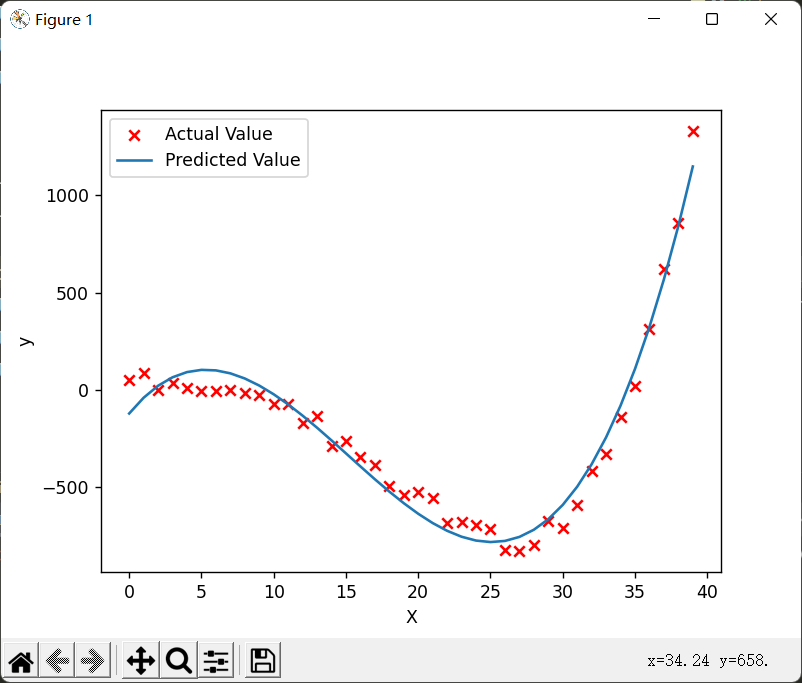展开全文机器学习 python • 线性回归是基本的统计和机器学习技术之一。经济，计算机科学，社会科学等等学科中，无论是统计分析，或者是机器学习，还是科学计算，都有很大的机会需要用到线性模型。建议先学习它，然后再尝试更复杂的方法。本文... 线性回归是基本的统计和机器学习技术之一。经济，计算机科学，社会科学等等学科中，无论是统计分析，或者是机器学习，还是科学计算，都有很大的机会需要用到线性模型。建议先学习它，然后再尝试更复杂的方法。 本文主要介绍如何逐步在Python中实现线性回归。而至于线性回归的数学推导、线性回归具体怎样工作，参数选择如何改进回归模型将在以后说明。 ## 回归 回归分析是统计和机器学习中最重要的领域之一。有许多可用的回归方法。线性回归就是其中之一。而线性回归可能是最重要且使用最广泛的回归技术之一。这是最简单的回归方法之一。它的主要优点之一是线性回归得到的结果十分容易解释。那么回归主要有： • 简单线性回归 • 多元线性回归 • 多项式回归 ## 如何在python中实现线性回归 ### 用到的packages • NumPy NumPy是Python的基础科学软件包，它允许在单维和多维数组上执行许多高性能操作。 • scikit-learn scikit-learn是在NumPy和其他一些软件包的基础上广泛使用的Python机器学习库。它提供了预处理数据，减少维数，实现回归，分类，聚类等的方法。 • statsmodels 如果要实现线性回归并且需要功能超出scikit-learn的范围，则应考虑使用statsmodels可以用于估算统计模型，执行测试等。 ### scikit-learn的简单线性回归 ### 1.导入用到的packages和类 import numpy as np from sklearn.linear_model import LinearRegression 2.创建数据 x = np.array([5, 15, 25, 35, 45, 55]).reshape((-1, 1)) y = np.array([5, 20, 14, 32, 22, 38]) 现在就生成了两个数组:输入x(回归变量)输出y(预测变量)，来看看 >>> print(x) [[ 5]     ] >>> print(y) [ 5 20 14 32 22 38] 可以看到x是二维的而y是一维的，因为在复杂一点的模型中，系数不只一个。这里就用到了.reshape()来进行转换。 3.建立模型 创建一个类的实例LinearRegression，它将代表回归模型： model = LinearRegression() 现在开始拟合模型，首先可以调用.fit()函数来得到优的 ₀和 ₁，具体有下面两种等价方法 model.fit(x, y) model = LinearRegression().fit(x, y) 4.查看结果 拟合模型之后就是查看与模型相关的各项参数 >>> r_sq = model.score(x, y) >>> print('coefficient of determination:', r_sq) coefficient of determination: 0.715875613747954 .score()函数可以获得模型的 ²，再看看系数 >>> print('intercept:', model.intercept_) intercept: 5.633333333333329 >>> print('slope:', model.coef_) slope: [0.54] 可以看到系数和截距分别为[0.54]和5.6333，注意系数是一个二维数组哦。 5.预测效果 一般而言，线性模型最后就是用来预测，我们来看下预测效果 >>> y_pred = model.predict(x) >>> print('predicted response:', y_pred, sep='n') predicted response: [ 8.33333333 13.73333333 19.13333333 24.53333333 29.93333333 35.33333333] 当然也可以使用下面的方法 >>> y_pred = model.intercept_ + model.coef_ * x >>> print('predicted response:', y_pred, sep='n') predicted response: [[ 8.33333333] [13.73333333] [19.13333333] [24.53333333] [29.93333333] [35.33333333]] 除了可以利用样本内的数据进行预测，也可以用样本外的数据进行预测。 >>> x_new = np.arange(5).reshape((-1, 1)) >>> print(x_new) [    ] >>> y_new = model.predict(x_new) >>> print(y_new) [5.63333333 6.17333333 6.71333333 7.25333333 7.79333333] 至此，一个简单的线性回归模型就建立起来了。 ## scikit-learn的多元线性回归 直接开始吧 1.导入包和类，并创建数据 import numpy as np from sklearn.linear_model import LinearRegression x = [[0, 1], [5, 1], [15, 2], [25, 5], [35, 11], [45, 15], [55, 34], [60, 35]] y = [4, 5, 20, 14, 32, 22, 38, 43] x, y = np.array(x), np.array(y) 看看数据 >>> print(x) [[ 0 1] [ 5 1] [15 2] [25 5] [35 11] [45 15] [55 34] [60 35]] >>> print(y) [ 4 5 20 14 32 22 38 43] 2.建立多元回归模型 model = LinearRegression().fit(x, y) 3.查看结果 >>> r_sq = model.score(x, y) >>> print('coefficient of determination:', r_sq) coefficient of determination: 0.8615939258756776 >>> print('intercept:', model.intercept_) intercept: 5.52257927519819 >>> print('slope:', model.coef_) slope: [0.44706965 0.25502548] 4.预测 #样本内 >>> y_pred = model.predict(x) >>> print('predicted response:', y_pred, sep='n') predicted response: [ 5.77760476 8.012953 12.73867497 17.9744479 23.97529728 29.4660957 38.78227633 41.27265006] #样本外 >>> x_new = np.arange(10).reshape((-1, 2)) >>> print(x_new) [[0 1] [2 3] [4 5] [6 7] [8 9]] >>> y_new = model.predict(x_new) >>> print(y_new) [ 5.77760476 7.18179502 8.58598528 9.99017554 11.3943658 ] 所有的结果都在结果里，就不再过多解释。再看看多项式回归如何实现。 ## 多项式回归 导入包和创建数据 import numpy as np from sklearn.linear_model import LinearRegression from sklearn.preprocessing import PolynomialFeatures x = np.array([5, 15, 25, 35, 45, 55]).reshape((-1, 1)) y = np.array([15, 11, 2, 8, 25, 32]) 多项式回归和之前不一样的是需要对数据转换，因为模型里包含 ²等变量，所以在创建数据之后要将x转换为 ²。 transformer = PolynomialFeatures(degree=2, include_bias=False) 再看看数据 >>> print(x_) [[ 5. 25.] [ 15. 225.] [ 25. 625.] [ 35. 1225.] [ 45. 2025.] [ 55. 3025.]] 建模 接下来的步骤就和之前的类似了。其实多项式回归只是多了个数据转换的步骤，因此从某种意义上，多项式回归也算是线性回归。 model = LinearRegression().fit(x_, y) 查看结果 >>> r_sq = model.score(x_, y) >>> print('coefficient of determination:', r_sq) coefficient of determination: 0.8908516262498564 >>> print('intercept:', model.intercept_) intercept: 21.372321428571425 >>> print('coefficients:', model.coef_) coefficients: [-1.32357143 0.02839286] 预测 >>> y_pred = model.predict(x_) >>> print('predicted response:', y_pred, sep='n') predicted response: [15.46428571 7.90714286 6.02857143 9.82857143 19.30714286 34.46428571] 那么本次多项式回归的所有结果都在上面了，一目了然。 =================================================================== 欢迎关注我的专栏： 早起的学习小站​zhuanlan.zhihu.com公-众-号：早起python 展开全文python 回归 • sklearn实现多元线性回归及多项式回归，个人学习笔记，简单易懂，实现多项式回归sklearn • 多元线性回归 上一个博客 我们说了一元线性回归，那么来看一下多元线性回归 一元函数的公式是 而多元函数的公式： 其实就是相当于位置参数的变量都增多了，我们的解决办法依旧可以使用我们一元线性回归当中的代价... # 多元线性和多项式回归 上一个博客 我们说了一元线性回归，那么来看一下多元线性回归 一元函数的公式是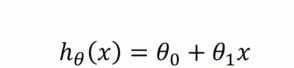而多元函数的公式：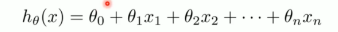其实就是相当于位置参数的变量都增多了，我们的解决办法依旧可以使用我们一元线性回归当中的代价函数和梯度下降算法。 代价函数依旧是：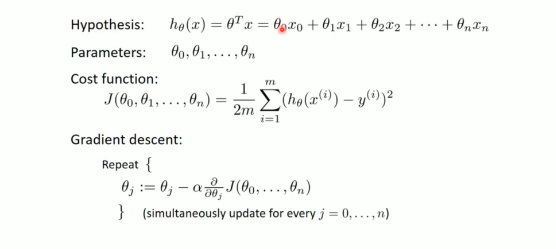梯度下降算法为： 我们可以看到，有多少个参数变量，我们就都给他构造出来，只是比一元线性回归中多一些参数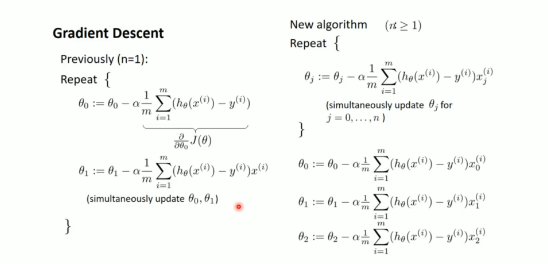直接上代码： #### 先导入包： import numpy as np from numpy import genfromtxt import matplotlib.pyplot as plt from mpl_toolkits.mplot3d import Axes3D  最后一个包是构造3D图像所需要导入的包 #### 处理数据: # 读入数据 data = genfromtxt(r"Delivery.csv",delimiter=',') print(data) # 切分数据 x_data = data[:,:-1] # 相当于坐标 y_data = data[:,-1] # 相当于label print(x_data) print(y_data)  #### 构造代价函数和梯度下降算法: # 设置学习率 lr = 0.0001 # 设置参数 theta0 = 0 theta1 = 0 theta2 = 0 #最大迭代次数 epochs = 1000 # 设置代价函数 def compute_error(theta0,theta1,theta2,x_data,t_data): totalerror = 0 for i in range(0,len(x_data)): totalerror += (y_data[i] - (theta0 + theta1 * x_data[i,0] + theta2 * x_data[i,1])) ** 2 return totalerror/float(len(x_data)) def gradient_descent_runner(x_data, y_data, theta0, theta1, theta2, lr, epochs): m = float(len(x_data)) for i in range(epochs): theta0_1 = 0 theta1_1 = 0 theta2_1 = 0 for j in range(0,len(x_data)): theta0_1 += (1/m) * ((theta0 + theta1 * x_data[j,0] + theta2 * x_data[j,1]) - y_data[j]) theta1_1 += (1/m) * (x_data[j,0]) * ((theta0 + theta1 * x_data[j,0] + theta2 * x_data[j,1]) - y_data[j]) theta2_1 += (1/m) * (x_data[j,1]) * ((theta0 + theta1 * x_data[j,0] + theta2 * x_data[j,1]) - y_data[j]) theta0 = theta0 - (lr*theta0_1) theta1 = theta1 - (lr*theta1_1) theta2 = theta2 - (lr*theta2_1) return theta0, theta1, theta2  #### 最后使用函数回归画出我们想要的图形： theta0, theta1, theta2 = gradient_descent_runner(x_data, y_data, theta0, theta1, theta2, lr, epochs) ax = plt.figure().add_subplot(111,projection = '3d') ax.scatter(x_data[:,0],x_data[:,1],y_data,c='r',marker='o',s=100) x0 = x_data[:,0] x1 = x_data[:,1] x0,x1 = np.meshgrid(x0,x1) z = theta0 + theta1 * x0 + theta2 * x1 ax.plot_surface(x0,x1,z) ax.set_xlabel('Miles') ax.set_ylabel('Num of Deliveries') ax.set_zlabel('Time') plt.show()  我们最后画出来的3D模型为：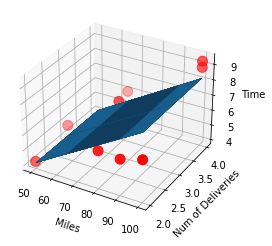用sklearn 实现： #### 导入包: import numpy as np from numpy import genfromtxt from sklearn import linear_model import matplotlib.pyplot as plt from mpl_toolkits.mplot3d import Axes3D  #### 读取并处理数据： # 读入数据 data = genfromtxt(r"Delivery.csv",delimiter=',') print(data) # 切分数据 x_data = data[:,:-1] y_data = data[:,-1]  #### 创建模型 model = linear_model.LinearRegression() model.fit(x_data, y_data)  此时model.intercept_存储的相当于截距参数，而model.coef_存储的是含参系数值 #### 画图 ax = plt.figure().add_subplot(111, projection = '3d') ax.scatter(x_data[:,0], x_data[:,1], y_data, c = 'r', marker = 'o', s = 100) #点为红色三角形 x0 = x_data[:,0] x1 = x_data[:,1] # 生成网格矩阵 x0, x1 = np.meshgrid(x0, x1) # z = model.intercept_ + x0*model.coef_ + x1*model.coef_ # 画3D图 ax.plot_surface(x0, x1, model.predict(x0,x1)) #设置坐标轴 w ax.set_xlabel('Miles') ax.set_ylabel('Num of Deliveries') ax.set_zlabel('Time') #显示图像 plt.show()  # 多项式回归 我们之前所构造出来的都是直线或者是超平面，但是对于下面的散点集，我们使用直线就不会非常吻合的构造出所有点所要表达的趋势，所以我们这里需要的是一个多项式表达的曲线或者超曲面。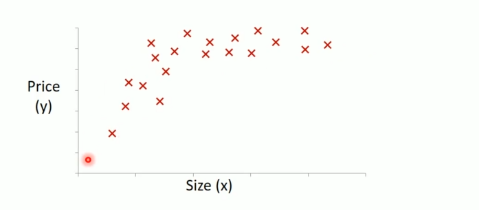曲线所达到的效果更好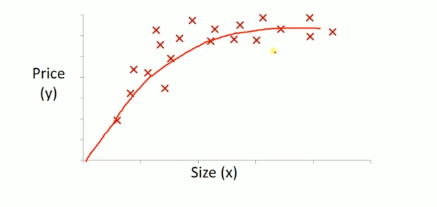所以我们构造是形如下面的函数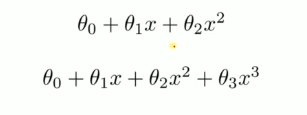对于多项式问题我们直接使用sklearn运行代码： #### 导入包: import numpy as np import matplotlib.pyplot as plt from sklearn.preprocessing import PolynomialFeatures from sklearn.linear_model import LinearRegression  #### 导入并处理数据： data = np.genfromtxt("job.csv", delimiter=",") x_data = data[1:,1] y_data = data[1:,2] x_data = x_data[:,np.newaxis] y_data = y_data[:,np.newaxis]  #### 创建并拟合模型 model = LinearRegression() model.fit(x_data, y_data)  #### 此时画出的图像为：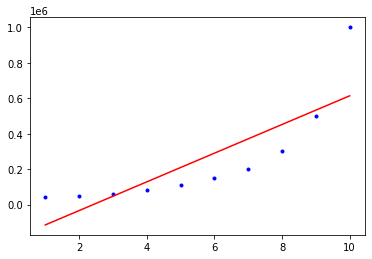是一元线性的直线，那么我们想要曲线来表示的话： # 定义多项式回归,degree的值可以调节多项式的特征 poly_reg = PolynomialFeatures(degree=9) # 特征处理在这里插入图片描述 x_poly = poly_reg.fit_transform(x_data) # 定义回归模型 lin_reg = LinearRegression() # 训练模型 lin_reg.fit(x_poly, y_data)  再次画图： # 画图 plt.plot(x_data, y_data, 'b.') plt.plot(x_data, lin_reg.predict(poly_reg.fit_transform(x_data)), c='r') # poly_reg.fit_transform(x_data) 预测前必须得做特征处理 plt.title('Truth or Bluff (Polynomial Regression)') plt.xlabel('Position level') plt.ylabel('Salary') plt.show()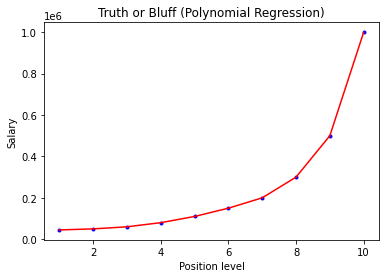这个曲线是由一段一段的线段组成的，如果我们想要连续曲折圆滑的曲线就需要np.linspace生成等间隔数列这样就比较圆滑了。 plt.plot(x_data, y_data, 'b.') x_test = np.linspace(1,10,100) x_test = x_test[:,np.newaxis] plt.plot(x_test, lin_reg.predict(poly_reg.fit_transform(x_test)), c='r') plt.title('Truth or Bluff (Polynomial Regression)') plt.xlabel('Position level') plt.ylabel('Salary') plt.show()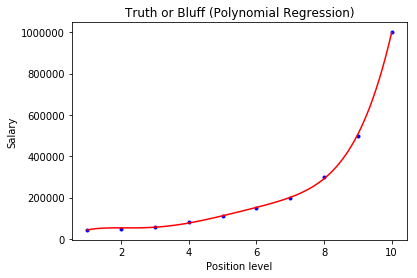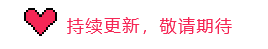展开全文• 1、多元线性回归 n 个预测器变量，那么模型可以用以下方程表示： 波士顿房价数据集。该数据集包含 506 座房子的 13 个特征，均值为$1000。你将用一个模型拟合这 13 个特征，以预测房价 from sklearn.linear_model ...机器学习
• python多项式回归Video Link 影片连结 You can view the code used in this Episode here: SampleCode 您可以在此处查看 此剧 集中使用的代码： SampleCode 导入我们的数据 (Importing our Data) The first step ...python
•  # 画3D图 ax.plot_surface(x0, x1, z) #设置坐标轴 ax.set_xlabel('Miles') ax.set_ylabel('Num of Deliveries') ax.set_zlabel('Time') #显示图像 plt.show() 多项式回归 例子 import numpy as np import ...sklearn 线性回归 python
• sklearn实现多项式线性回归_一元/多元 【Python机器学习系列（八）】1. 多项式一元回归2. 多项式多元回归机器学习 python sklearn 多项式 线性回归
• 多项式回归是一种线性回归形式，其中自变量x和因变量y之间的关系被建模为n次多项式。多项式回归拟合x的值与y的相应条件均值之间的非线性关系，表示为E(y | x)为什么多项式回归：研究人员假设的某些关系是曲线的。...
• 多项式回归也称多元非线性回归，是指包含两个以上变量的非线性回归模型。对于多元非线性回归模型求解的传统解决方案，仍然是想办法把它转化成标准的线性形式的多元回归模型来处理。 多元非线性回归分析方程如果自...
• 主要为大家详细介绍了PyTorch搭建多项式回归模型，具有一定的参考价值，感兴趣的小伙伴们可以参考一下
• python多项式回归Let’s start with an example. We want to predict the Price of a home based on the Area and Age. The function below was used to generate Home Prices and we can pretend this is “real-...
•python 机器学习
• 1. 多元线性回归基本假定与表达式 1.1 基本假定 1.2 具体值的表达方式 1.3 向量表达形式 1.4 矩阵表达方式 2. 多元线性回归求解 2.1 矩阵解 2.2 代数解 2.3 几何解 2.3.1 线性方程组的几何意义 2.3.2 最小二乘法 2.4...线性回归
• ## 多项式回归

万次阅读 多人点赞 2018-10-02 23:08:37
多项式回归 多项式回归，回归函数是回归变量多项式的回归。多项式回归模型是线性回归模型的一种，此时回归函数关于回归系数是线性的。由于任一函数都可以用多项式逼近，因此多项式回归有着广泛应用。 直线回归研究...多项式回归 Scipy
•机器学习 python
• 目录 1、概述 1.1 有监督学习 1.2 多项式回归 2 概念 3 案例实现——方法1 ...1.2 多项式回归 ...上一次我们讲解了线性回归，...多项式回归(Polynomial Regression)是研究一个因变量与一 个或多个自变量间多项式的...人工智能 python
• 线性回归的改进版本中的多项式回归。如果您知道线性回归，那么对您来说很简单。如果没有，我将在本文中解释这些公式。还有其他先进且更有效的机器学习算法。但是，学习基于线性的回归技术是一个好主意。因为它们简单...python 机器学习 人工智能
• 多项式 将参数和训练数据都作为向量来处理，可以使计算变得更简单。 由于训练数据有很多，所以我们把1 行数据当作1 个训练数据，以矩阵的形式来处理会更好。 矩阵与参数向量θ 的积如下 ...机器学习 python 人工智能
• 看完这篇文章，将会：1.使用scikit-learn实现多项式回归 2.创建一个模型，训练它，测试它，并使用它机器学习 python
• 这节我们会介绍多项式回归和在机器学习中最常遇到的问题过拟合.欠拟合 主要介绍使用模型正则化来解决过拟合的问题, 1.多项式回归 之前学习过线性回归的，我们学习多项式回归会很简单.我们遇到的很多都是非线性的...sklearn 机器学习
•线性回归
• 代码如下： import numpy as np from sklearn.linear_model import LinearRegression from sklearn.metrics import mean_squared_error import matplotlib.pyplot as plt from sklearn.preprocessing import ...
• 本文介绍回归模型的原理知识，包括线性回归、多项式回归和逻辑回归，并详细介绍Python Sklearn机器学习库的LinearRegression和LogisticRegression算法及回归分析实例。进入基础文章，希望对您有所帮助。机器学习 线性回归...